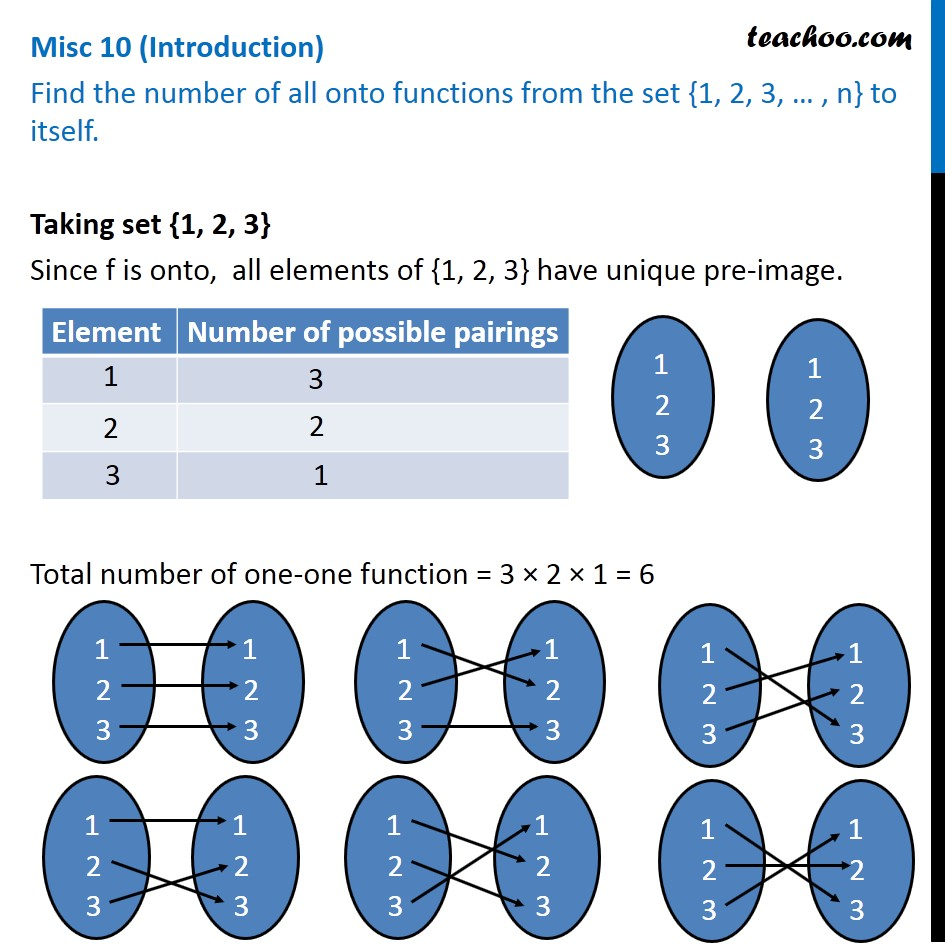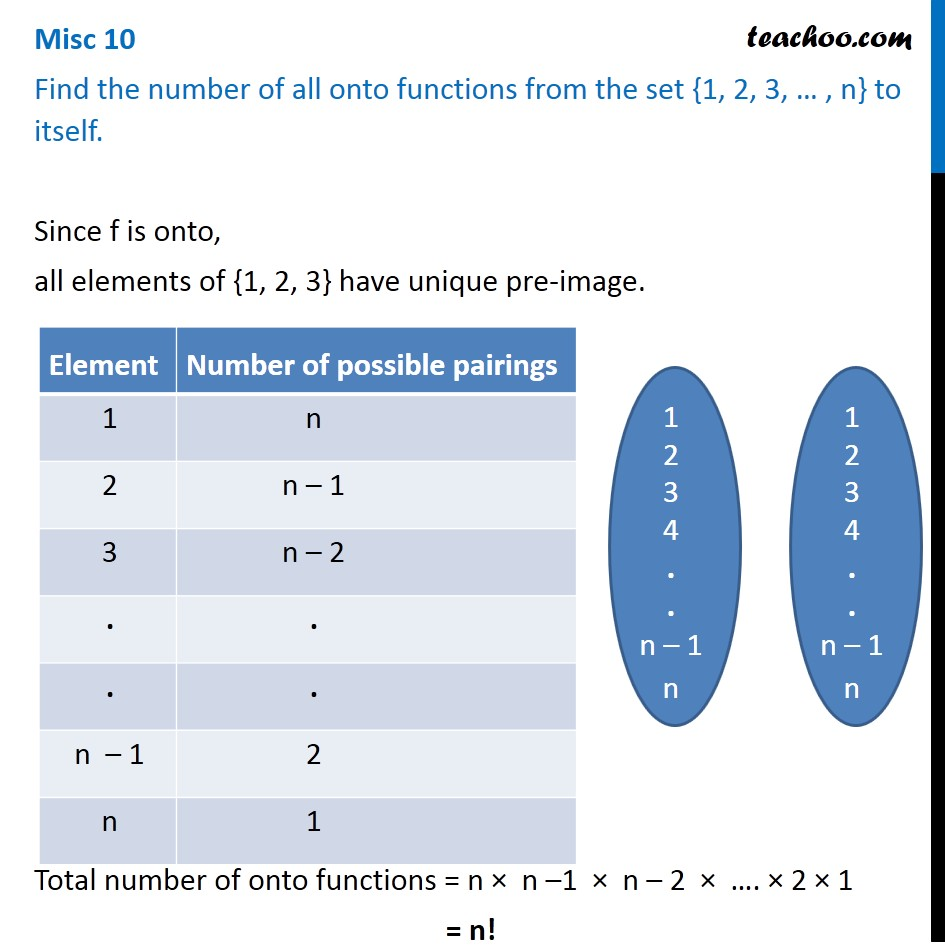Miscellaneous

Chapter 1 Class 12 Relation and Functions (Term 1)
Serial order wise### Transcript

Misc 10 (Introduction) Find the number of all onto functions from the set {1, 2, 3, … , n} to itself. Taking set {1, 2, 3} Since f is onto, all elements of {1, 2, 3} have unique pre-image. Total number of one-one function = 3 × 2 × 1 = 6 Misc 10 Find the number of all onto functions from the set {1, 2, 3, … , n} to itself. Since f is onto, all elements of {1, 2, 3} have unique pre-image. Total number of onto functions = n × n –1 × n – 2 × …. × 2 × 1 = n!